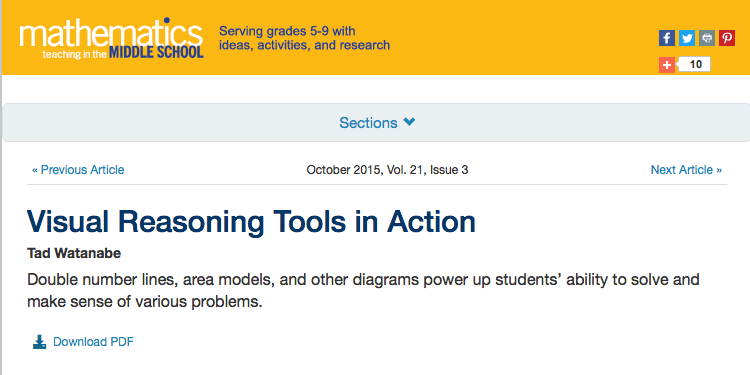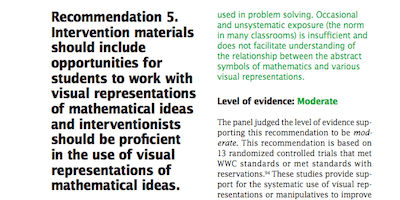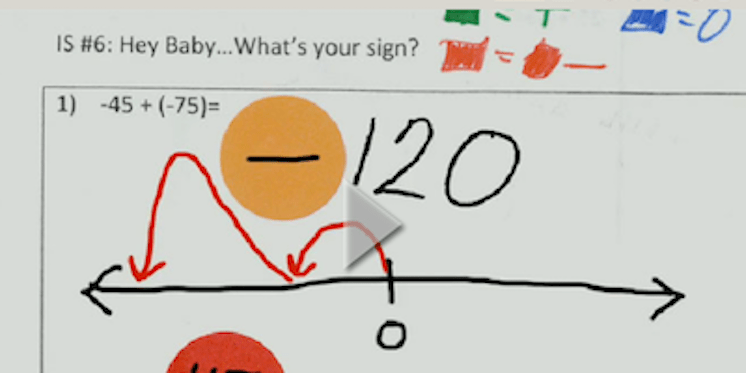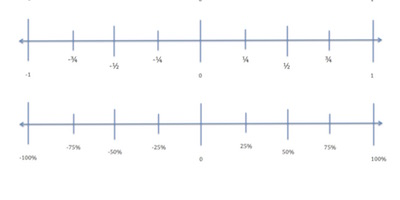Number Lines

Number lines are a specific type of visual representation. They can be used to enhance students' visual reasoning with numbers and investigate a variety of numerical relationships. In addition to comparing rational numbers, students in the middle grades can also use the number line to understand the value of and density of numbers between various units.

Field Guide Alignment: Math and Math Interventions, Principle 3

PROFESSIONAL DEVELOPMENT

Learn about the strategyBuilding the Number Line

Online PD module
Developed by Annenberg LearnerVisual Reasoning Tools in Action

Article
Developed by Ted WatanabeTraveling from Arithmetic to Algebra

Article
Developed by Joy DarleyPractice guide
Developed by IES

VIDEOS

See the strategy in actionTeacher demonstration
Developed by the Teaching ChannelDemo lesson using number lines
Developed by the Teaching Channel

INSTRUCTIONAL MATERIALS

Use the strategyElevator Arithmetic

Lesson plan
Developed by NCTMMath Shorts: Absolute Value

Video demonstration and student activity
Developed by PBS LearningMediaAlgebra on a Number Line

Lesson plan
Developed by NCTMRational Numbers and Football

Online student activity
Developed by PBS LearningMediaLearning Mathematics Through Representations - LMR

Lesson plans
Developed by Culture CognitionNumber Lines

Printable resource
Developed by MSM